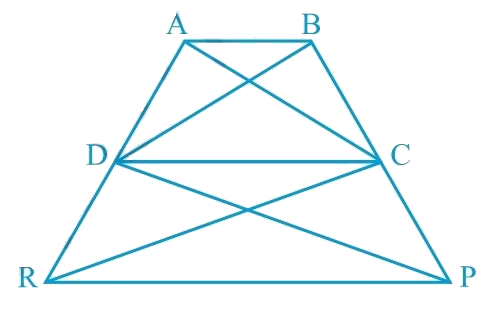# Ex.9.3 Q16 Areas of Parallelograms and Triangles Solution - NCERT Maths Class 9

Go back to  'Ex.9.3'

## Question

In the given figure,

$$ar (DRC)$$ $$= ar (DPC)$$ and $$ar (BDP) = ar (ARC)$$.

Show that both the quadrilaterals $$ABCD$$ and $$DCPR$$ are trapeziums.

Video Solution
Areas Of Parallelograms And Triangles
Ex 9.3 | Question 16

## Text Solution

What is known?

$$ar (DRC) = ar (DPC)$$ and $$ar (BDP) = ar (ARC).$$

What is unknown?

How we can show that quadrilaterals $$ABCD$$ and $$DCPR$$ are trapeziums.

Reasoning:

We know that if Two triangles having the same base (or equal bases) and equal areas lie between the same parallels.

Steps:

It is given that

$$\text{Area} (\Delta DRC )= \text{Area} (\Delta DPC )$$As $$\Delta DRC$$ and $$\Delta DPC$$ lie on the same base $$DC$$ and have equal areas, therefore, they must lie between the same parallel lines.

According to Theorem $$9.3$$ : Two triangles having the same base (or equal bases) and equal areas lie between the same parallels.

$\therefore {{DC || RP}}$

Therefore, $$DCPR$$ is a trapezium.

It is also given that

\begin{align}\text { Area }(\Delta {BDP})=\text { Area }(\Delta {ARC})\end{align}

Area $$(\Delta \, BDP)$$ - Area $$(\Delta\, DPC)$$ = Area $$(\triangle \,ARC)$$ -Area $$(\Delta \, DRC)$$

\begin{align} {\therefore \text { Area }(\Delta {BDC})}&={\text { Area }(\Delta {ADC})}\end{align}

Since \begin{align} (\Delta BDC )\, and \,(\Delta ADC)\end{align} are on the same base $$CD$$ and have equal areas, they must lie between the same parallel lines.

According to Theorem $$9.3$$ : Two triangles having the same base (or equal bases) and equal areas lie between the same parallels.

\begin{align}\therefore \quad A B \| C D\end{align}

Therefore, $$ABCD$$ is a trapezium.

Video Solution
Areas Of Parallelograms And Triangles
Ex 9.3 | Question 16